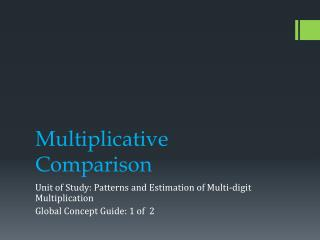Download PresentationMultiplicative Comparison

# Multiplicative Comparison - PowerPoint PPT PresentationDownload Presentation## Multiplicative Comparison

- - - - - - - - - - - - - - - - - - - - - - - - - - - E N D - - - - - - - - - - - - - - - - - - - - - - - - - - -
##### Presentation Transcript

1. Multiplicative Comparison Unit of Study: Patterns and Estimation of Multi-digit Multiplication Global Concept Guide: 1 of 2

2. Notice the increase in complexity of problems from Day 1 to Day 2 Day 1 Content Development Day 2 Go Math TE 45A Go Math TE p. 49

3. Day 3 of this GCG is allotted for differentiated instruction. Use the EQ and resources on the Enrich/Reteach/Intervention slide for Day 3 instruction.

4. Day 1 • EQ: How can you represent comparison problems? • Engage students by using the “Unlock the Problem” on TE p. 45. Use the Teach and Talk to help facilitate discussion to ensure students understand how the bar model represents the problem and how to use the model to write and solve an equation. • Use the H.O.T. question on p. 45 to distinguish multiplicative comparison from additive comparison. • Translating a comparison sentence into an equation and vice versa is an important skill for understanding and solving real-world problems. Have students practice this contextualizing and decontextualizing through the Lesson 2.1 problems referenced in GCG. • By the end of Day 1 students should be able to use bar models to represent comparison problems and translate the model into an equation. • Evidence of learning: Jayden’s Pear Tree

5. Day 2 • EQ: How does a bar model help you solve multiplicative and additive comparison problems? • Engage: Students solve Go Math “Unlock the Problem: Example 1” on TE p. 49. Challenge students to use what they have learned about drawing models to help them solve the problem. Allow students to struggle as they try to work through the problem before you offer them support through questioning. See Teach and Talk Go Math TE p. 49 for scaffolding questions. • Students should work with a partner to solve #1 on TE p. 50. • Use HOT questions in the GCG to facilitate whole group discussion. • Pose “Unlock the Problem: Example 2” on TE p. 50 for students to use a bar model to solve. Facilitate discussion to compare and contrast this additive comparison problem to the earlier multiplicative comparison problems. • Students should work with a partner to complete #2 and 3 on Go Math SE p. 51, and 11 on p. 52. • By the end of Day 2, students should be able to use bar models to solve multiplicative and additive comparison problems. • Evidence of learning: Draw a bar model to solve #4 on Go Math SE p. 51.

6. Day 3 • EQ: How does a bar model help you solve multiplicative and additive comparison problems? • Re-teach: • Re-teach page – R9 • Model Me Math - Provide students with counters to model comparison problems prior to drawing bar model • Core: • Enrich page – E9 – Multiplication Match- Up • Model Me Math – additional comparison problems • Enrich: • SE p. 51 #7, SE p. 52 #10 • Students can create multiplicative and additive comparison problems. • Enrich page – E10 – Mixed Models • Evidence of learning: Unit 2 Performance Task, “Friendly Finches” Enrich/Reteach/Intervention